SNOS966Q May   2001  – September 2014

PRODUCTION DATA.

1. Features
2. Applications
3. Description
4. Revision History
5. Description (continued)
6. Pin Configuration and Functions
7. Specifications
8. Detailed Description
9. Application and Implementation
1. 9.1 Application Information
2. 9.2 Typical Application
10. 10Power Supply Recommendations
11. 11Layout
12. 12Device and Documentation Support
13. 13Mechanical, Packaging, and Orderable Information

• D|8
• DBV|5
• D|8

## 7 Specifications

### 7.1 Absolute Maximum Ratings(1)(2)

over operating free-air temperature range (unless otherwise noted)
MIN MAX UNIT
VIN Differential ±2.5 V
Output Short Circuit Duration See (4) and (6)
Supply Voltage (V+ - V) 13.5 V
Voltage at Input/Output pins V+ +0.8
V −0.8
V
Input Current ±10 mA
Junction Temperature(5) +150 °C
Soldering Information Infrared or Convection Reflow (20 sec) 235 °C
Wave Soldering Lead Temp.(10 sec) 260 °C
(1) Absolute Maximum Ratings indicate limits beyond which damage to the device may occur. Operating Ratings indicate conditions for which the device is intended to be functional, but specific performance is not ensured. For ensured specifications and the test conditions, see the Electrical Characteristics.
(2) If Military/Aerospace specified devices are required, please contact the TI Sales Office/Distributors for availability and specifications.
(3) Human body model, 1.5 kΩ in series with 100 pF. Machine Model, 0 Ω in series with 200 pF.
(4) Applies to both single-supply and split-supply operation. Continuous short circuit operation at elevated ambient temperature can result in exceeding the maximum allowed junction temperature of 150°C.
(5) The maximum power dissipation is a function of TJ(MAX), RθJA, and TA. The maximum allowable power dissipation at any ambient temperature is PD = (TJ(MAX) - TA)/ RθJA . All numbers apply for packages soldered directly onto a PC board.

### 7.2 Handling Ratings

MIN MAX UNIT
Tstg Storage temperature range −65 +150 °C
V(ESD) Electrostatic discharge(3) Human body model (HBM), per ANSI/ESDA/JEDEC JS-001, all pins(1) 2000 V
Machine model (MM)(3) 200
Charged device model (CDM), per JEDEC specification JESD22-C101, all pins(2) 1000
(1) JEDEC document JEP155 states that 2000-V HBM allows safe manufacturing with a standard ESD control process.
(2) JEDEC document JEP157 states that 1000-V CDM allows safe manufacturing with a standard ESD control process.
(3) JEDEC document JEP157 states that 200-V MM allows safe manufacturing with a standard ESD control process.

### 7.3 Recommended Operating Conditions(1)

over operating free-air temperature range (unless otherwise noted)
MIN MAX UNIT
Supply Voltage (V+ – V) 2.7 12.8 V
Operating Temperature Range(2) −40 +85 °C
(1) Absolute Maximum Ratings indicate limits beyond which damage to the device may occur. Operating Ratings indicate conditions for which the device is intended to be functional, but specific performance is not ensured. For ensured specifications and the test conditions, see the Electrical Characteristics.
(2) The maximum power dissipation is a function of TJ(MAX), RθJA, and TA. The maximum allowable power dissipation at any ambient temperature is PD = (TJ(MAX) - TA)/ RθJA. All numbers apply for packages soldered directly onto a PC board.

### 7.4 Thermal Information

THERMAL METRIC(1) LMH6642 LMH6643 LMH6644 UNIT
DBV05A D08A DGK08A D14A PW14A
5 PINS 8 PINS 8 PINS 14 PINS 14 PINS
RθJA Junction-to-ambient Thermal Resistance(2) 265 190 235 145 155 °C/W

### 7.5 3V Electrical Characteristics

Unless otherwise specified, all limits ensured for V+ = 3V, V = 0V, VCM = VO = V+/2, VID (input differential voltage) as noted (where applicable) and RL = 2kΩ to V+/2.
PARAMETER TEST CONDITIONS AT
TEMPERATURE
EXTREMES
V+ = 3V, V = 0V,
VCM = VO = V+/2, VID
RL = 2 kΩ to V+/2
UNIT
MIN TYP MAX MIN(1) TYP(2) MAX(1)
BW −3dB BW AV = +1, VOUT = 200mVPP 80 115 MHz
AV = +2, −1, VOUT = 200mVPP 46
BW0.1dB 0.1dB Gain Flatness AV = +2, RL = 150Ω to V+/2,
Rf = 402Ω, VOUT = 200mVPP
19 MHz
PBW Full Power Bandwidth AV = +1, −1dB, VOUT = 1VPP 40 MHz
en Input-Referred Voltage Noise f = 100kHz 17 nV/√Hz
f = 1kHz 48
in Input-Referred Current Noise f = 100kHz 0.90 pA/√Hz
f = 1kHz 3.3
THD Total Harmonic Distortion f = 5MHz, VO = 2VPP, AV = −1,
RL = 100Ω to V+/2
−48 dBc
DG Differential Gain VCM = 1V, NTSC, AV = +2
RL =150Ω to V+/2
0.17%
RL =1kΩ to V+/2 0.03%
DP Differential Phase VCM = 1V, NTSC, AV = +2
RL =150Ω to V+/2
0.05 deg
RL =1kΩ to V+/2 0.03
CT Rej. Cross-Talk Rejection f = 5MHz, Receiver:
Rf = Rg = 510Ω, AV = +2
47 dB
TS Settling Time VO = 2VPP, ±0.1%, 8pF Load,
VS = 5V
68 ns
SR Slew Rate (3) AV = −1, VI = 2VPP 90 120 V/µs
VOS Input Offset Voltage For LMH6642 and LMH6644 ±7 ±1 ±5 mV
For LMH6643 ±7 ±1 ±3.4
TC VOS Input Offset Average Drift See (4) ±5 µV/°C
IB Input Bias Current See (5) −3.25 −1.50 −2.60 µA
IOS Input Offset Current 1000 20 800 nA
RIN Common Mode Input Resistance 3
CIN Common Mode Input Capacitance 2 pF
CMVR Input Common-Mode Voltage Range CMRR ≥ 50dB −0.1 −0.5 −0.2 V
1.6 1.8 2.0
CMRR Common Mode Rejection Ratio VCM Stepped from 0V to 1.5V 72 95 dB
AVOL Large Signal Voltage Gain VO = 0.5V to 2.5V
RL = 2kΩ to V+/2
75 80 96 dB
VO = 0.5V to 2.5V
RL = 150Ω to V+/2
70 74 82
VO Output Swing
High
RL = 2kΩ to V+/2, VID = 200mV 2.90 2.98 V
RL = 150Ω to V+/2, VID = 200mV 2.80 2.93
Output Swing
Low
RL = 2kΩ to V+/2, VID = −200mV 25 75 mV
RL = 150Ω to V+/2, VID = −200mV 75 150
ISC Output Short Circuit Current Sourcing to V+/2
VID = 200mV (6)
35 50 95 mA
Sinking to V+/2
VID = −200mV (6)
40 55 110
IOUT Output Current VOUT = 0.5V from either supply ±65 mA
+PSRR Positive Power Supply Rejection Ratio V+ = 3.0V to 3.5V, VCM = 1.5V 75 85 dB
IS Supply Current (per channel) No Load 4.50 2.70 4.00 mA
(1) All limits are ensured by testing or statistical analysis.
(2) Typical values represent the most likely parametric norm.
(3) Slew rate is the average of the rising and falling slew rates.
(4) Offset voltage average drift determined by dividing the change in VOS at temperature extremes by the total temperature change.
(5) Positive current corresponds to current flowing into the device.
(6) Short circuit test is a momentary test. See Note 7 under 5 V Electrical Characteristics.

### 7.6 5V Electrical Characteristics

Unless otherwise specified, all limits ensured for V+ = 5V, V = 0V, VCM = VO = V+/2, VID (input differential voltage) as noted (where applicable) and RL = 2kΩ to V+/2.
PARAMETER TEST CONDITIONS AT TEMPERATURE EXTREMES V+ = 5V, V = 0V,
VCM = VO = V+/2, VID
RL = 2kΩ to V+/2
UNIT
MIN TYP MAX MIN(1) TYP(2) MAX(1)
BW −3dB BW AV = +1, VOUT = 200mVPP 90 120 MHz
AV = +2, −1, VOUT = 200mVPP 46
BW0.1dB 0.1dB Gain Flatness AV = +2, RL = 150Ω to V+/2,
Rf = 402Ω, VOUT = 200mVPP
15 MHz
PBW Full Power Bandwidth AV = +1, −1dB, VOUT = 2VPP 22 MHz
en Input-Referred Voltage Noise f = 100kHz 17 nV/√Hz
f = 1kHz 48
in Input-Referred Current Noise f = 100kHz 0.90 pA/√Hz
f = 1kHz 3.3
THD Total Harmonic Distortion f = 5MHz, VO = 2VPP, AV = +2 −60 dBc
DG Differential Gain NTSC, AV = +2
RL =150Ω to V+/2
0.16%
RL = 1kΩ to V+/2 0.05%
DP Differential Phase NTSC, AV = +2
RL = 150Ω to V+/2
0.05 deg
RL = 1kΩ to V+/2 0.01
CT Rej. Cross-Talk Rejection f = 5MHz, Receiver:
Rf = Rg = 510Ω, AV = +2
47 dB
TS Settling Time VO = 2VPP, ±0.1%, 8pF Load 68 ns
SR Slew Rate (3) AV = −1, VI = 2VPP 95 125 V/µs
VOS Input Offset Voltage For LMH6642 and LMH6644 ±7 ±1 ±5 mV
For LMH6643 ±7 ±1 ±3.4
TC VOS Input Offset Average Drift See (4) ±5 µV/°C
IB Input Bias Current See (5) −3.25 −1.70 −2.60 µA
IOS Input Offset Current 1000 20 800 nA
RIN Common Mode Input Resistance 3
CIN Common Mode Input Capacitance 2 pF
CMVR Input Common-Mode Voltage Range CMRR ≥ 50dB −0.1 −0.5 −0.2 V
3.6 3.8 4.0
CMRR Common Mode Rejection Ratio VCM Stepped from 0V to 3.5V 72 95 dB
AVOL Large Signal Voltage Gain VO = 0.5V to 4.50V
RL = 2kΩ to V+/2
82 86 98 dB
VO = 0.5V to 4.25V
RL = 150Ω to V+/2
72 76 82
VO Output Swing
High
RL = 2kΩ to V+/2, VID = 200mV 4.90 4.98 V
RL = 150Ω to V+/2, VID = 200mV 4.65 4.90
Output Swing
Low
RL = 2kΩ to V+/2, VID = −200mV 25 100 mV
RL = 150Ω to V+/2, VID = −200mV 100 150
ISC Output Short Circuit Current Sourcing to V+/2
VID = 200mV (6)(7)
40 55 115 mA
Sinking to V+/2
VID = −200mV (6)(7)
55 70 140
IOUT Output Current VO = 0.5V from either supply ±70 mA
+PSRR Positive Power Supply Rejection Ratio V+ = 4.0V to 6V 79 90 dB
IS Supply Current (per channel) No Load 5.00 2.70 4.25 mA
(1) All limits are ensured by testing or statistical analysis.
(2) Typical values represent the most likely parametric norm.
(3) Slew rate is the average of the rising and falling slew rates.
(4) Offset voltage average drift determined by dividing the change in VOS at temperature extremes by the total temperature change.
(5) Positive current corresponds to current flowing into the device.
(6) Short circuit test is a momentary test. See Note 7.
(7) Output short circuit duration is infinite for VS < 6V at room temperature and below. For VS > 6V, allowable short circuit duration is 1.5ms.

### 7.7 ±5V Electrical Characteristics

Unless otherwise specified, all limits ensured for V+ = 5V, V = −5V, VCM = VO = 0V, VID (input differential voltage) as noted (where applicable) and RL = 2kΩ to ground.
PARAMETER TEST CONDITIONS AT TEMPERATURE EXTREMES V+ = 5V, V = −5V,
VCM = VO = 0V, VID
UNIT
MIN TYP MAX MIN(2) TYP(1) MAX(2)
BW −3dB BW AV = +1, VOUT = 200mVPP 95 130 MHz
AV = +2, −1, VOUT = 200mVPP 46
BW0.1dB 0.1dB Gain Flatness AV = +2, RL = 150Ω to V+/2,
Rf = 806Ω, VOUT = 200mVPP
12 MHz
PBW Full Power Bandwidth AV = +1, −1dB, VOUT = 2VPP 24 MHz
en Input-Referred Voltage Noise f = 100kHz 17 nV/√Hz
f = 1kHz 48
in Input-Referred Current Noise f = 100kHz 0.90 pA/√Hz
f = 1kHz 3.3
THD Total Harmonic Distortion f = 5MHz, VO = 2VPP, AV = +2 −62 dBc
DG Differential Gain NTSC, AV = +2
RL = 150Ω to V+/2
0.15%
RL = 1kΩ to V+/2 0.01%
DP Differential Phase NTSC, AV = +2
RL = 150Ω to V+/2
0.04 deg
RL = 1kΩ to V+/2 0.01
CT Rej. Cross-Talk Rejection f = 5MHz, Receiver:
Rf = Rg = 510Ω, AV = +2
47 dB
TS Settling Time VO = 2VPP, ±0.1%, 8pF Load,
VS = 5V
68 ns
SR Slew Rate (4) AV = −1, VI = 2VPP 100 135 V/µs
VOS Input Offset Voltage For LMH6642 and LMH6644 ±7 ±1 ±5 mV
For LMH6643 ±7 ±1 ±3.4
TC VOS Input Offset Average Drift See (7) ±5 µV/°C
IB Input Bias Current See (3) −3.25 −1.60 −2.60 µA
IOS Input Offset Current 1000 20 800 nA
RIN Common Mode Input Resistance 3
CIN Common Mode Input Capacitance 2 pF
CMVR Input Common-Mode Voltage Range CMRR ≥ 50dB −5.1 −5.5 −5.2 V
3.6 3.8 4.0
CMRR Common Mode Rejection Ratio VCM Stepped from −5V to 3.5V 74 95 dB
AVOL Large Signal Voltage Gain VO = −4.5V to 4.5V,
RL = 2kΩ
84 88 96 dB
VO = −4.0V to 4.0V,
RL = 150Ω
74 78 82
VO Output Swing
High
RL = 2kΩ, VID = 200mV 4.90 4.96 V
RL = 150Ω, VID = 200mV 4.65 4.80
Output Swing
Low
RL = 2kΩ, VID = −200mV −4.96 −4.90 V
RL = 150Ω, VID = −200mV −4.80 −4.65
ISC Output Short Circuit Current Sourcing to Ground
VID = 200mV (5)(6)
35 60 115 mA
Sinking to Ground
VID = −200mV (5)(6)
65 85 145
IOUT Output Current VO = 0.5V from either supply ±75 mA
PSRR Power Supply Rejection Ratio (V+, V) = (4.5V, −4.5V) to (5.5V, −5.5V) 78 90 dB
IS Supply Current (per channel) No Load 5.50 2.70 4.50 mA
(1) Typical values represent the most likely parametric norm.
(2) All limits are ensured by testing or statistical analysis.
(3) Positive current corresponds to current flowing into the device.
(4) Slew rate is the average of the rising and falling slew rates.
(5) Short circuit test is a momentary test. See (6).
(6) Output short circuit duration is infinite for VS < 6V at room temperature and below. For VS > 6V, allowable short circuit duration is 1.5ms.
(7) Offset voltage average drift determined by dividing the change in VOS at temperature extremes by the total temperature change.

### 7.8 Typical Performance Characteristics

V+ = +5, V = −5V, RF = RL = 2 kΩ. Unless otherwise specified.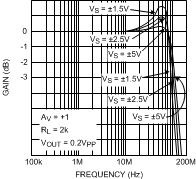Figure 1. Closed Loop Frequency Response
for Various Supplies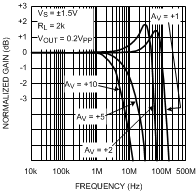Figure 3. Closed Loop Gain vs. Frequency
for Various Gain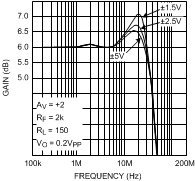Figure 5. Closed Loop Gain vs. Frequency
for Various Supplies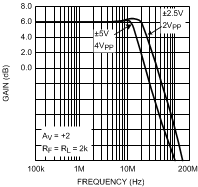Figure 7. Large Signal Frequency ResponseFigure 9. Closed Loop Frequency Response
for Various Supplies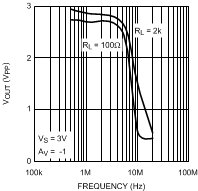Figure 11. VOUT (VPP) for THD < 0.5%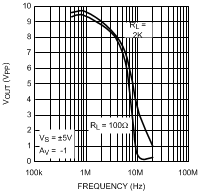Figure 13. VOUT (VPP) for THD < 0.5%Figure 15. Open Loop Gain/Phase
for Various Temperature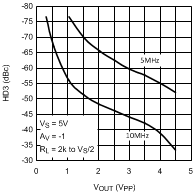Figure 17. HD3 (dBc) vs. Output Swing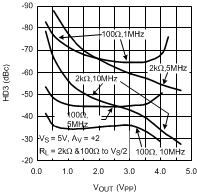Figure 19. HD3 vs. Output Swing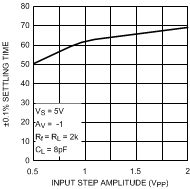Figure 21. Settling Time vs. Input Step Amplitude
(Output Slew and Settle Time)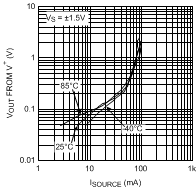Figure 23. VOUT from V+ vs. ISOURCE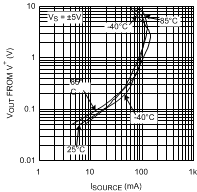Figure 25. VOUT from V+ vs. ISOURCE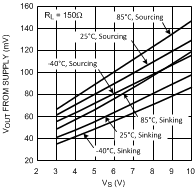Figure 27. Swing vs. VS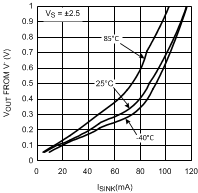Figure 29. Output Sinking Saturation Voltage vs. IOUT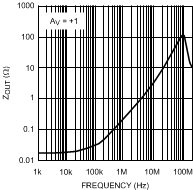Figure 31. Closed Loop Output Impedance
vs. Frequency AV = +1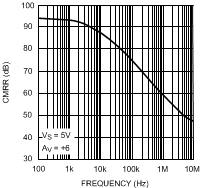Figure 33. CMRR vs. Frequency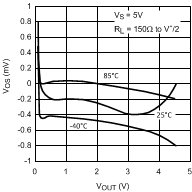Figure 35. VOS vs. VOUT (Typical Unit)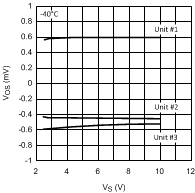Figure 37. VOS vs. VS (for 3 Representative Units)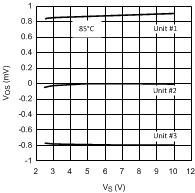Figure 39. VOS vs. VS (for 3 Representative Units)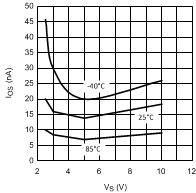Figure 41. IOS vs. VS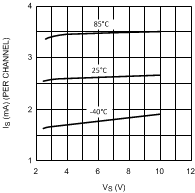Figure 43. IS vs. VS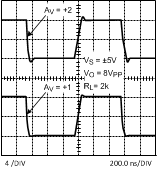Figure 45. Large Signal Step Response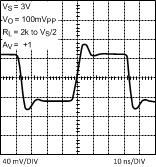Figure 47. Small Signal Step Response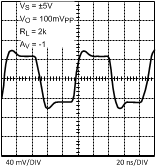Figure 49. Small Signal Step Response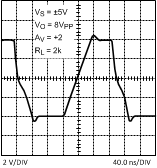Figure 51. Large Signal Step Response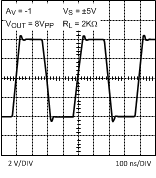Figure 53. Large Signal Step Response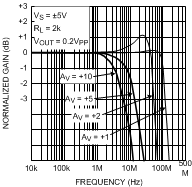Figure 2. Closed Loop Gain vs. Frequency
for Various GainFigure 4. Closed Loop Frequency Response
for Various TemperatureFigure 6. Closed Loop Frequency Response
for Various Temperature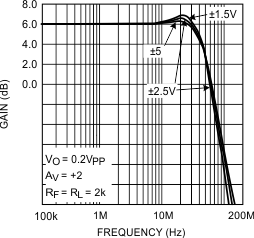Figure 8. Closed Loop Small Signal Frequency Response
for Various Supplies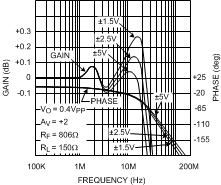Figure 10. ±0.1dB Gain Flatness
for Various Supplies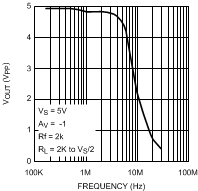Figure 12. VOUT (VPP) for THD < 0.5%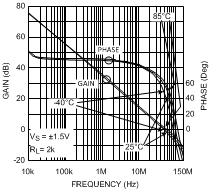Figure 14. Open Loop Gain/Phase
for Various Temperature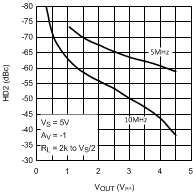Figure 16. HD2 (dBc) vs. Output SwingFigure 18. HD2 vs. Output Swing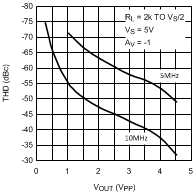Figure 20. THD (dBc) vs. Output Swing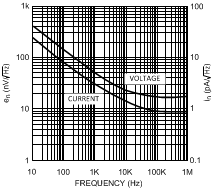Figure 22. Input Noise vs. Frequency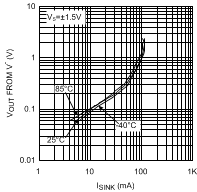Figure 24. VOUT from V vs. ISINK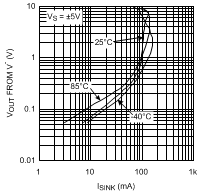Figure 26. VOUT from V vs. ISINK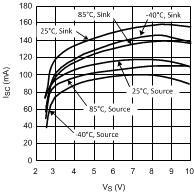Figure 28. Short Circuit Current (to VS/2) vs. VS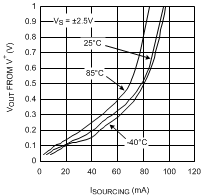Figure 30. Output Sourcing Saturation Voltage vs. IOUT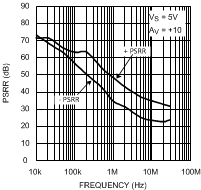Figure 32. PSRR vs. Frequency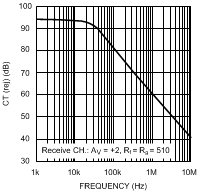Figure 34. Crosstalk Rejection vs. Frequency
(Output to Output)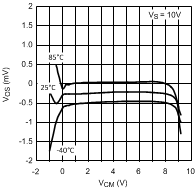Figure 36. VOS vs. VCM (Typical Unit)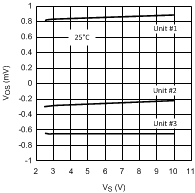Figure 38. VOS vs. VS (for 3 Representative Units)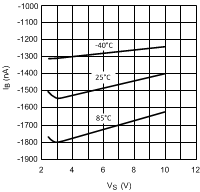Figure 40. IB vs. VS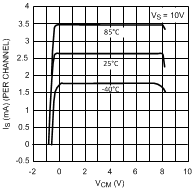Figure 42. IS vs. VCM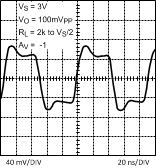Figure 44. Small Signal Step Response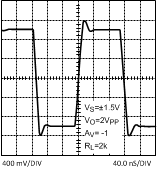Figure 46. Large Signal Step ResponseFigure 48. Small Signal Step Response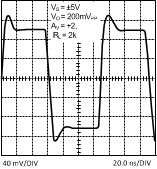Figure 50. Small Signal Step Response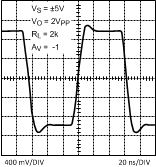Figure 52. Large Signal Step Response